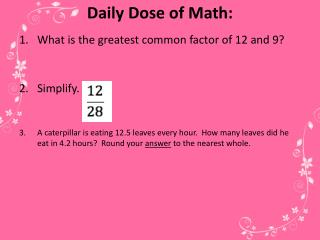DownloadDownload PresentationDaily Dose of Math:

# Daily Dose of Math:

Télécharger la présentation## Daily Dose of Math:

- - - - - - - - - - - - - - - - - - - - - - - - - - - E N D - - - - - - - - - - - - - - - - - - - - - - - - - - -
##### Presentation Transcript

1. Daily Dose of Math: • What is the greatest common factor of 12 and 9? • Simplify. • A caterpillar is eating 12.5 leaves every hour. How many leaves did he eat in 4.2 hours? Round your answer to the nearest whole.

2. Comparing Fractions Similar Fractions: have the same denominator! common denominators! lowest common denominators! * My goal is... to use knowledge of the least common multiple and lowest common denominator to compare and order fractions.

3. Comparing Fractions Similar Fractions: have the same denominator! common denominators! lowest common denominators! * My goal is... to use knowledge of the least common multiple and lowest common denominator to compare and order fractions.

4. Comparing Fractions Similar Fractions: have the same denominator! common denominators! lowest common denominators! * My goal is... to use knowledge of the least common multiple and lowest common denominator to compare and order fractions.

5. Comparing Fractions Similar Fractions: have the same denominator! common denominators! lowest common denominators! * My goal is... to use knowledge of the least common multiple and lowest common denominator to compare and order fractions.

6. Least Common Multiple, or LCM, is the smallest multiple two or more numbers have in common. • The multiples of a number are all products that result in multiplying that number with the counting numbers.